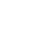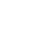LV. 1
GP 12# 【心得】徹甲3傷害上限的壹些測試和心得

GP27 BP-

TL；DR：壹把弩的徹三傷害上限大致為 武器版面真實傷害*2.04*0.24+-0.5

（以下數值使用真實傷害計算-重弩/1.5 輕弩/1.3，所有傷害數值皆是打樁傷害）

（24%在不同的怪和不同怪的狀態下會浮動，因此有時可以打出超出木樁傷害的數字）

（300+150）*.24=108+1.5=110

（300+20+150）*.24=113+1.5=115

（300+150）*1.15*.24=124+1.5=126

（300+20+150）*1.15*.24=129+1.5=131

（300+x+150)1.15=600 x=72

600*.24 應該能達到144+1.5=146，而實際只能達到144，不知道是因為系統省略了太多的小數點還是有收益遞減，反正就是少了，雖然已經很接近了但是對與數據黨來說是很遺憾的。

（300+x+150)1.15=610 x=80

LV. 17
GP 1k2 樓 knn knnzero
GP0 BP-

（300+x+150)1.15=610 x=80

(300+x+90)1.15=610 x=140.4

face基於日前微軟官方表示 Internet Explorer 不再支援新的網路標準，可能無法使用新的應用程式來呈現網站內容，在瀏覽器支援度及網站安全性的雙重考量下，為了讓巴友們有更好的使用體驗，巴哈姆特即將於 2019年9月2日 停止支援 Internet Explorer 瀏覽器的頁面呈現和功能。